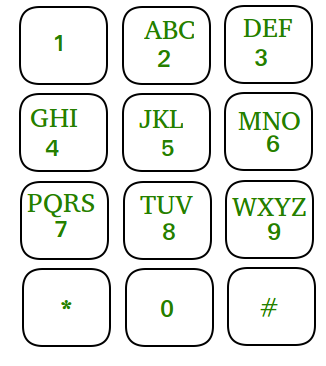GeeksforGeeks App
Open AppBrowser
Continue

# Count of distinct Numbers that can be formed by chess knight in N moves on a mobile keypad

Given an integer N and a chess knight placed in mobile keypad. The task is to count the total distinct N digit numbers which can be formed by the chess knight with N moves. As the answer can be very large give the value of answer modulo 109 + 7.

Note: In each move a chess knight can move 2 units horizontally and one unit vertically or two units vertically and one unit horizontally.

A demo mobile keypad is shown in image where ‘*’ and ‘#’ are not considered as part of a number.Examples:

Input: N = 1
Output: 10
Explanation: Placing the knight over any numeric cell of the 10 cells is sufficient.

Input: N = 2
Output: 20
Explanation: All the valid number are [04, 06, 16, 18, 27, 29, 34, 38, 40, 43, 49, 60, 61, 67, 72, 76, 81, 83, 92, 94]

Approach: The idea is to find the possible cells that can be reached from a given cell for every cell and add all of them to find the answer. Follow the steps below to solve the problem:

• Initialize the vector v[10, 1], and temp.
• Iterate over the range [1, N) using the variable i and perform the following tasks:
• Find the values for all cells in temp[] and then store them in vector v[].
• Initialize the variable sum as 0 to store the answer.
• Iterate over the range [0, 10) using the variable i and perform the following tasks:
• Add the value of v[i] to the variable sum.
• After performing the above steps, print the value of sum as the answer.

Below is the implementation of the above approach.

## C++

 `// C++ program for the above approach``#include ``using` `namespace` `std;` `// Function to find the total number of ways``int` `knightCalling(``int` `N)``{``    ``int` `mod = 1000000007;` `    ``// Base Case``    ``if` `(N == 1)``        ``return` `10;``    ``vector<``int``> v(10, 1);``    ``vector<``int``> temp(10);` `    ``// No cell can be reached from a``    ``// cell with value 5``    ``v = 0;``    ``for` `(``int` `i = 1; i < N; i++)``    ``{``      ` `        ``// Find the possible values from all cells``        ``temp = (v + v) % mod;``        ``temp = (v + v) % mod;``        ``temp = (v + v) % mod;``        ``temp = (v + v) % mod;``        ``temp = (v + v + v) % mod;``        ``temp = (v + v + v) % mod;``        ``temp = (v + v) % mod;``        ``temp = (v + v) % mod;``        ``temp = (v + v) % mod;` `        ``// Store them``        ``for` `(``int` `j = 0; j < 10; j++)``            ``v[j] = temp[i];``    ``}` `    ``// Find the answer``    ``int` `sum = 0;``    ``for` `(``int` `i = 0; i < 10; i++)``        ``sum = (sum + v[i]) % mod;``    ``return` `sum;``}` `// Driver Code``int` `main()``{``    ``int` `N = 2;``    ``cout << knightCalling(N);``}` `// This code is contributed by Samim Hossain Mondal.`

## Java

 `// Java program for the above approach``import` `java.util.*;``class` `GFG{` `// Function to find the total number of ways``static` `int` `knightCalling(``int` `N)``{``    ``int` `mod = ``1000000007``;` `    ``// Base Case``    ``if` `(N == ``1``)``        ``return` `10``;``    ``int` `[]v = ``new` `int``[``10``];``    ``int` `[]temp = ``new` `int``[``10``];``    ``Arrays.fill(v, ``1``);` `    ``// No cell can be reached from a``    ``// cell with value 5``    ``v[``5``] = ``0``;``    ``for` `(``int` `i = ``1``; i < N; i++)``    ``{``      ` `        ``// Find the possible values from all cells``        ``temp[``0``] = (v[``4``] + v[``6``]) % mod;``        ``temp[``1``] = (v[``6``] + v[``8``]) % mod;``        ``temp[``2``] = (v[``7``] + v[``9``]) % mod;``        ``temp[``3``] = (v[``4``] + v[``8``]) % mod;``        ``temp[``4``] = (v[``0``] + v[``3``] + v[``9``]) % mod;``        ``temp[``6``] = (v[``0``] + v[``1``] + v[``7``]) % mod;``        ``temp[``7``] = (v[``2``] + v[``6``]) % mod;``        ``temp[``8``] = (v[``1``] + v[``3``]) % mod;``        ``temp[``9``] = (v[``2``] + v[``4``]) % mod;` `        ``// Store them``        ``for` `(``int` `j = ``0``; j < ``10``; j++)``            ``v[i] = temp[i];``    ``}` `    ``// Find the answer``    ``int` `sum = ``0``;``    ``for` `(``int` `i = ``0``; i < ``10``; i++)``        ``sum = (sum + v[i]) % mod;``    ``return` `sum;``}` `// Driver Code``public` `static` `void` `main(String[] args)``{``    ``int` `N = ``2``;``    ``System.out.print(knightCalling(N));``}``}` `// This code is contributed by 29AjayKumar`

## Python3

 `# Python 3  program for the above approach` `# Function to find the total number of ways``def` `knightCalling(N):` `    ``mod ``=` `1000000007` `    ``# Base Case``    ``if` `(N ``=``=` `1``):``        ``return` `10``    ``v ``=` `[``1``]``*``10``    ``temp ``=` `[``0``]``*``10` `    ``# No cell can be reached from a``    ``# cell with value 5``    ``v[``5``] ``=` `0``    ``for` `i ``in` `range``(``1``, N):``      ` `        ``# Find the possible values from all cells``        ``temp[``0``] ``=` `(v[``4``] ``+` `v[``6``]) ``%` `mod``        ``temp[``1``] ``=` `(v[``6``] ``+` `v[``8``]) ``%` `mod``        ``temp[``2``] ``=` `(v[``7``] ``+` `v[``9``]) ``%` `mod``        ``temp[``3``] ``=` `(v[``4``] ``+` `v[``8``]) ``%` `mod``        ``temp[``4``] ``=` `(v[``0``] ``+` `v[``3``] ``+` `v[``9``]) ``%` `mod``        ``temp[``6``] ``=` `(v[``0``] ``+` `v[``1``] ``+` `v[``7``]) ``%` `mod``        ``temp[``7``] ``=` `(v[``2``] ``+` `v[``6``]) ``%` `mod``        ``temp[``8``] ``=` `(v[``1``] ``+` `v[``3``]) ``%` `mod``        ``temp[``9``] ``=` `(v[``2``] ``+` `v[``4``]) ``%` `mod` `        ``# Store them``        ``for` `j ``in` `range``(``10``):``            ``v[j] ``=` `temp[j]` `    ``# Find the answer``    ``sum` `=` `0``    ``for` `i ``in` `range``(``10``):``        ``sum` `=` `(``sum` `+` `v[i]) ``%` `mod``    ``return` `sum` `# Driver Code``if` `__name__ ``=``=` `"__main__"``:` `    ``N ``=` `2``    ``print``(knightCalling(N))` `    ``# This code is contributed by ukasp.`

## C#

 `// C# program for the above approach``using` `System;``class` `GFG{` `  ``// Function to find the total number of ways``  ``static` `int` `knightCalling(``int` `N)``  ``{``    ``int` `mod = 1000000007;` `    ``// Base Case``    ``if` `(N == 1)``      ``return` `10;``    ``int` `[]v = ``new` `int``;``    ``int` `[]temp = ``new` `int``;``    ``for``(``int` `i = 0; i < 10; i++) {``      ``v[i] = 1;``    ``}` `    ``// No cell can be reached from a``    ``// cell with value 5``    ``v = 0;``    ``for` `(``int` `i = 1; i < N; i++)``    ``{` `      ``// Find the possible values from all cells``      ``temp = (v + v) % mod;``      ``temp = (v + v) % mod;``      ``temp = (v + v) % mod;``      ``temp = (v + v) % mod;``      ``temp = (v + v + v) % mod;``      ``temp = (v + v + v) % mod;``      ``temp = (v + v) % mod;``      ``temp = (v + v) % mod;``      ``temp = (v + v) % mod;` `      ``// Store them``      ``for` `(``int` `j = 0; j < 10; j++)``        ``v[j] = temp[i];``    ``}` `    ``// Find the answer``    ``int` `sum = 0;``    ``for` `(``int` `i = 0; i < 10; i++)``      ``sum = (sum + v[i]) % mod;``    ``return` `sum;``  ``}` `  ``// Driver Code``  ``public` `static` `void` `Main()``  ``{``    ``int` `N = 2;``    ``Console.Write(knightCalling(N));``  ``}``}` `// This code is contributed by Samim Hossain Mondal.`

## Javascript

 ``

Output

`20`

Time Complexity: O(N)
Auxiliary Space: O(1)

My Personal Notes arrow_drop_up